• +91 9971497814
• info@interviewmaterial.com

# RD Chapter 23- The Straight Lines Ex-23.10 Interview Questions Answers

### Related Subjects

Question 1 :
Find the point of intersection of the following pairs of lines:
(i) 2x – y + 3 = 0 and x + y – 5 = 0
(ii) bx + ay = ab and ax + by = ab

(i) 2x – y + 3 = 0 and x +y – 5 = 0

Given:

The equations of thelines are as follows:

2x − y + 3 = 0 … (1)

x + y − 5 = 0 … (2)

Let us find the pointof intersection of pair of lines.

By solving (1) and (2)using cross – multiplication method, we get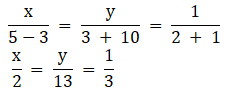x = 2/3 and y = 13/3

The point ofintersection is (2/3, 13/3)

(ii) bx + ay = ab and ax +by = ab

Given:

The equations of thelines are as follows:

bx + ay − ab = 0… (1)

ax + by = ab  ax + by − ab = 0 … (2)

Let us find the pointof intersection of pair of lines.

By solving (1) and (2)using cross – multiplication method, we get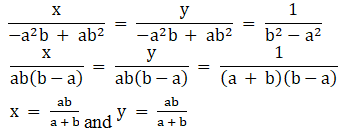The point ofintersection is (ab/a+b, ab/a+b)

Question 2 :

Find the coordinates of the vertices of a triangle, the equations ofwhose sides are: (i) x + y – 4 = 0, 2x – y + 3 0 and x – 3y + 2 = 0

(ii) y (t1 + t2) = 2x + 2at1t2,y (t2 + t3) = 2x + 2at2t3 and,y(t3 + t1) = 2x + 2at1t3.

(i) x + y – 4 = 0, 2x – y+ 3 0 and x – 3y + 2 = 0

Given:

x + y − 4 = 0, 2x − y+ 3 = 0 and x − 3y + 2 = 0

Let us find the pointof intersection of pair of lines.

x + y − 4 = 0 … (1)

2x − y + 3 = 0 … (2)

x − 3y + 2 = 0 … (3)

By solving (1) and (2)using cross – multiplication method, we get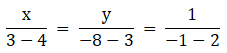x = 1/3, y = 11/3

Solving (1) and (3)using cross – multiplication method, we get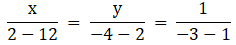x = 5/2, y = 3/2

Similarly, solving (2)and (3) using cross – multiplication method, we get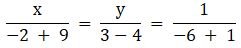x = – 7/5, y = 1/5

The coordinatesof the vertices of the triangle are (1/3, 11/3), (5/2, 3/2) and (-7/5, 1/5)

(ii) y (t1 +t2) = 2x + 2at1t2, y (t2 + t3)= 2x + 2at2t3 and, y(t3 + t1)= 2x + 2at1t3.

Given:

y (t1 +t2) = 2x + 2a t1t2, y (t2 + t3)= 2x + 2a t2t3 and y (t3 + t1)= 2x + 2a t1t3

Let us find the pointof intersection of pair of lines.

2x − y (t1 +t2) + 2a t1t2 = 0 … (1)

2x − y (t2 +t3) + 2a t2t3 = 0 … (2)

2x − y (t3 +t1) + 2a t1t3 = 0 … (3)

By solving (1) and (2)using cross – multiplication method, we get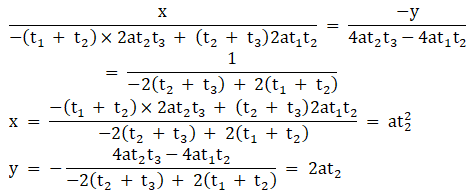Solving (1) and (3)using cross – multiplication method, we get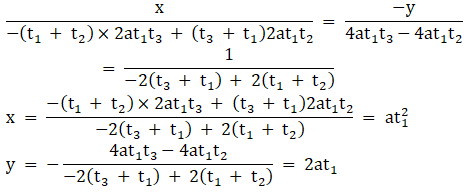Solving (2) and (3)using cross – multiplication method, we get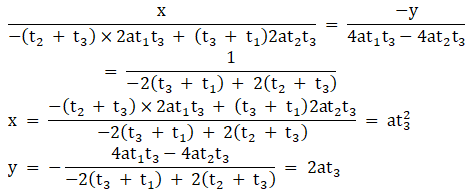The coordinatesof the vertices of the triangle are (at21, 2at1), (at22,2at2) and (at23, 2at3).

Question 3 :

Find the area of the triangle formed by the lines
y = m1x + c1, y = m2x + c2 andx = 0

Given:

y = m1x + c1 …(1)

y = m2x + c2 …(2)

x = 0 … (3)

In triangle ABC, letequations (1), (2) and (3) represent the sides AB, BC and CA, respectively.

Solving (1) and (2),we get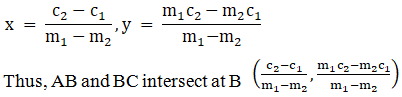Solving (1) and (3):

x = 0, y = c1

Thus, AB and CAintersect at A 0,c1.

Similarly, solving (2)and (3):

x = 0, y = c2

Thus, BC and CAintersect at C 0,c2.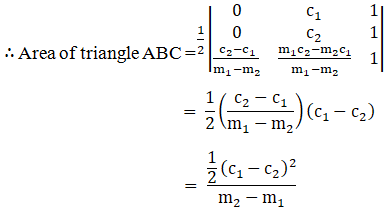Question 4 :

Find the equations of the medians of a triangle, the equations of whosesides are:
3x + 2y + 6 = 0, 2x – 5y + 4 = 0 and x – 3y – 6 = 0

Given:

3x + 2y + 6 = 0 … (1)

2x − 5y + 4 = 0 … (2)

x − 3y − 6 = 0 … (3)

Let us assume, intriangle ABC, let equations (1), (2) and (3) represent the sides AB, BC and CA,respectively.

Solving equations (1)and (2), we get

x = −2, y = 0

Thus, AB and BCintersect at B (−2, 0).

Now, solving (1) and(3), we get

x = – 6/11, y = –24/11

Thus, AB and CAintersect at A (-6/11, -24/11)

Similarly, solving (2)and (3), we get

x = −42, y = −16

Thus, BC and CAintersect at C (−42, −16).

Now, let D, E and F bethe midpoints the sides BC, CA and AB, respectively.

Then, we have: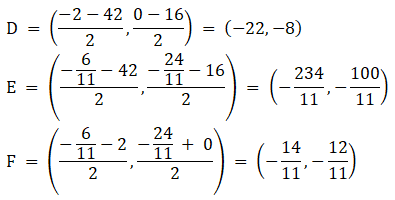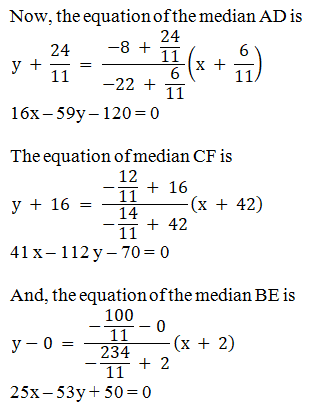The equations ofthe medians of a triangle are: 41x – 112y – 70 = 0,

16x – 59y – 120 = 0,25x – 53y + 50 = 0

Question 5 : Prove that the lines y = √3x + 1, y = 4 and y = -√3x + 2 form an equilateral triangle.

Given:

y = √3x + 1…… (1)

y = 4 ……. (2)

y = – √3x + 2……. (3)

Let us assume intriangle ABC, let equations (1), (2) and (3) represent the sides AB, BC and CA,respectively.

By solving equations(1) and (2), we get

x = √3, y = 4

Thus, AB and BCintersect at B(√3,4)

Now, solving equations(1) and (3), we get

x = 1/2√3, y = 3/2

Thus, AB and CAintersect at A (1/2√3, 3/2)

Similarly, solvingequations (2) and (3), we get

x = -2/√3, y = 4

Thus, BC and ACintersect at C (-2/√3,4)

Now, we have: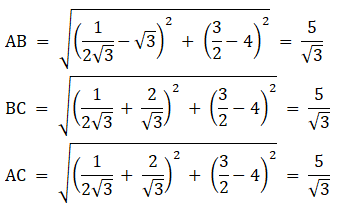Hence proved, thegiven lines form an equilateral triangle.

Todays Deals### RD Chapter 23- The Straight Lines Ex-23.10 Contributorskrishan

Name:
Email:

# Latest News# 9000 interview questions in different categories